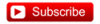# YouTubeT4-33: Fixed Income: Hedging the DV01

#### Nicole Seaman

##### Director of FRM Operations
Staff member
Subscriber
The DV01 is dollar change in the position for a one basis point (1 bps) decline in the interest rate (typically, yield). The DV01 is expressed per $100 face amount; for example,$0.035 implies that when rates drop by one basis point, the bond will increase in value by $0.035 per$100 face amount. So, if the face amount is $10.0 million, then a 1 bps decline anticipates an increase in value of$10,000,000 * 0.035/100 = +\$3,500.00. To hedge with instrument B (in this example, futures contracts), we use F(B) = F(A)*DV01(A)/DV01(B).Last edited: